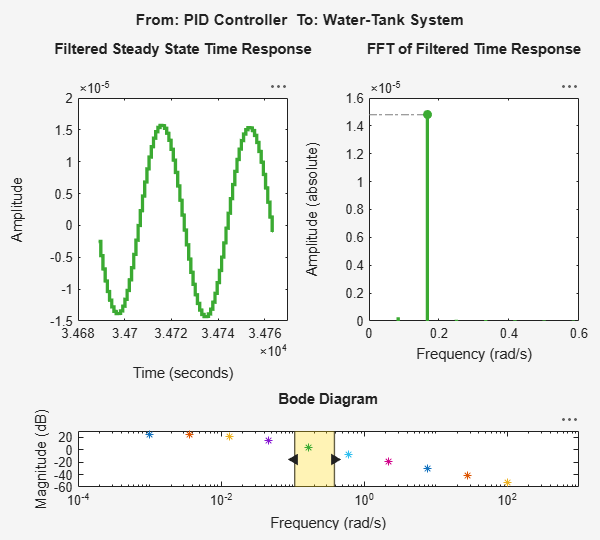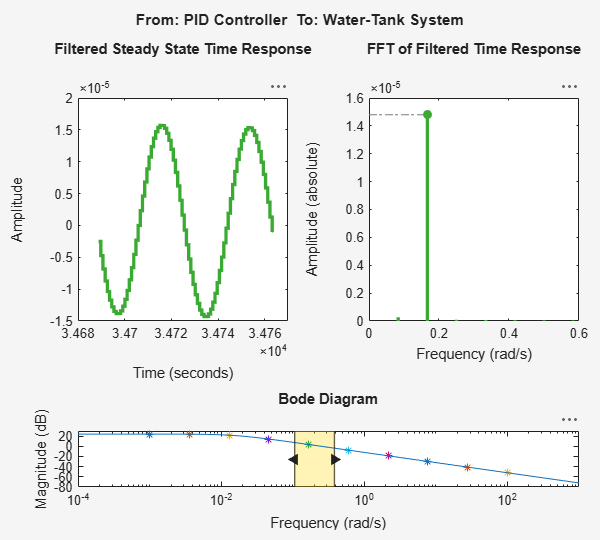# frest.simView

Package: frest

Plot frequency response model in time- and frequency-domain

## Syntax

```frest.simView(simout,input,sysest) frest.simView(simout,input,sysest,sys) ```

## Description

`frest.simView(simout,input,sysest)` plots the following frequency response estimation results:

• Time-domain simulation `simout` of the Simulink model

• FFT of time-domain simulation `simout`

• Bode of estimated system `sysest`

This Bode plot is available when you create the input signal using `frest.Sinestream` or `frest.Chirp`. In this plot, you can interactively select frequencies or a frequency range for viewing the results in all three plots.

You obtain `simout` and `sysest` from the `frestimate` command using the input signal `input`.

`frest.simView(simout,input,sysest,sys)` includes the linear system `sys` in the Bode plot when you create the input signal using `frest.Sinestream` or `frest.Chirp`. Use this syntax to compare the linear system to the frequency response estimation results.

## Examples

collapse all

The Simulation Results Viewer lets you examine the results of frequency response estimation frequency by frequency. You open the viewer using the `frest.simView` command. To do so, store the simulation data using the `simout` output argument of `frestimate`.

Estimate the open-loop response of the plant in the `watertank` model. First, open the model.

```model = 'watertank'; open_system(model) ```Define a linearization I/O set that specifies the plant, and find a steady-state operating point for estimation.

```io(1)=linio('watertank/PID Controller',1,'input'); io(2)=linio('watertank/Water-Tank System',1,'openoutput'); watertank_spec = operspec(model); opOpts = findopOptions('DisplayReport','off'); op = findop(model,watertank_spec,opOpts); ```

Then, create an input signal for estimation, and estimate the frequency response of the specified portion of the model. Use the `simout` output argument to store the estimation data.

```input = frest.Sinestream('Frequency',logspace(-3,2,10)); [sysest,simout] = frestimate(model,op,io,input); ```

Open the Simulation Results Viewer.

```frest.simView(simout,input,sysest) ```The viewer shows you the steady-state time response and the FFT of that response for all frequencies within the range you select on the Bode Diagram section of the viewer. These plots can help you identify when the response deviates from the expected response. For more information about using the Simulation Results Viewer, see Analyze Estimated Frequency Response.

If you have a linear model of the system you are estimating, you can use the model as a baseline response for comparison in the viewer. For instance, you can compare a model obtained by exact linearization to the estimated frequency response. Use the linearization I/O set and the operating point to compute an exact linearization of the `watertank` plant.

```syslin = linearize(model,io,op); ```

Open the Simulation Results Viewer again, this time providing `syslin` as an input argument.

```frest.simView(simout,input,sysest,syslin) ```The Bode Diagram section of the viewer includes a line showing the exact response `syslin`. This view can be useful to identify particular frequencies where the estimated response deviates from the linearization.

### Topics

Introduced in R2009b